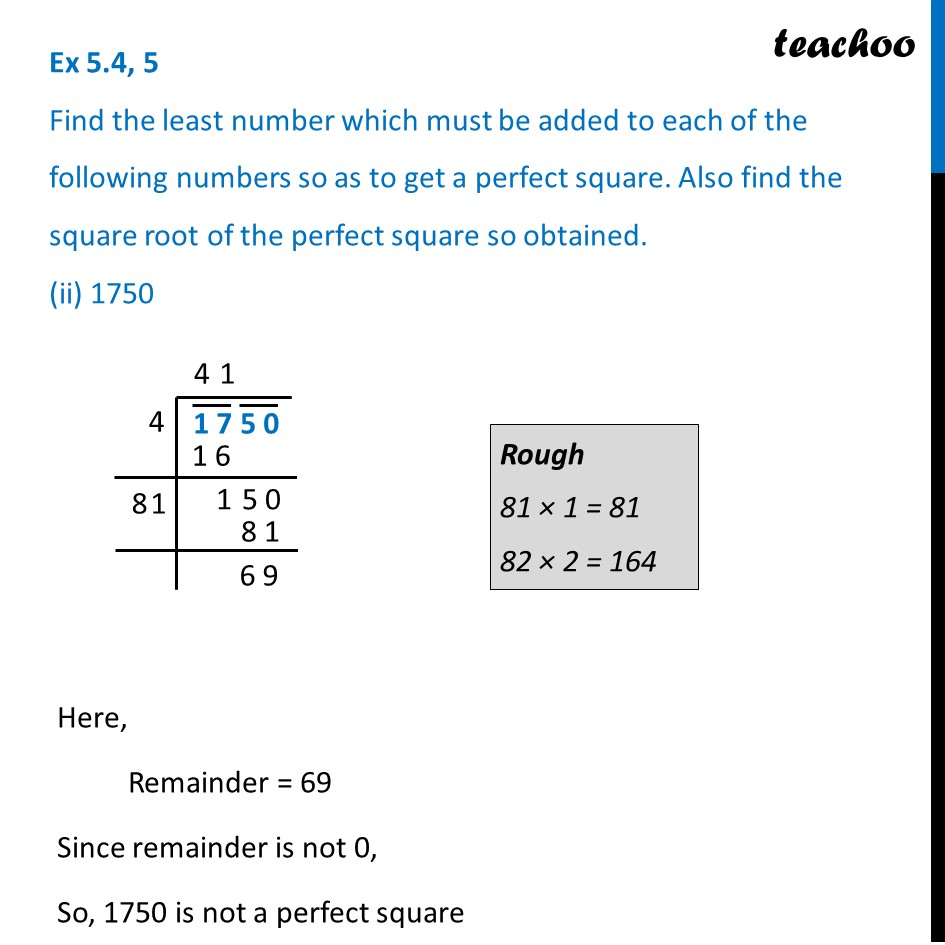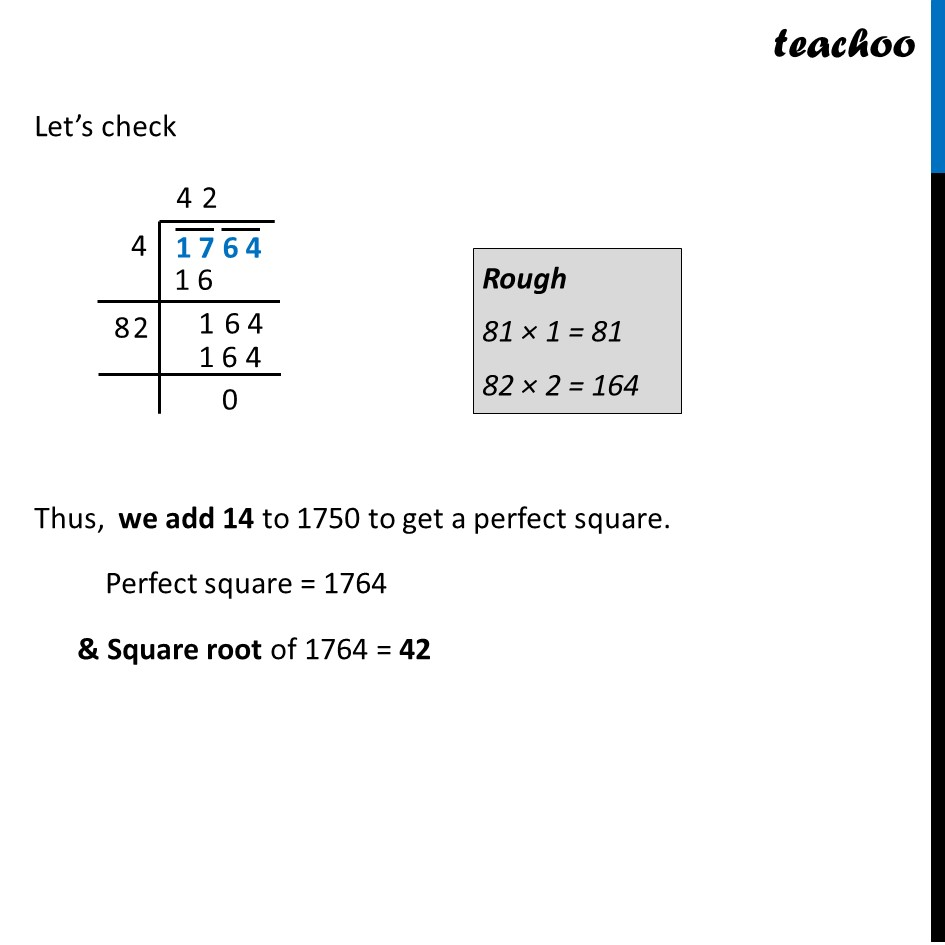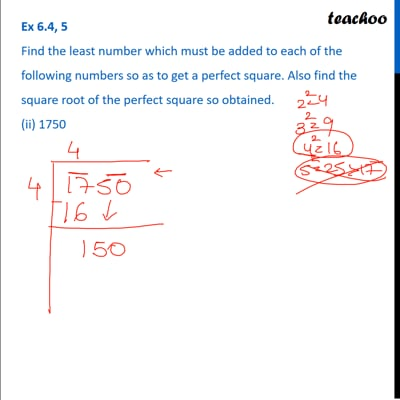Ex 5.4

Chapter 5 Class 8 Squares and Square Roots
Serial order wiseThis video is only available for Teachoo black users

Learn in your speed, with individual attention - Teachoo Maths 1-on-1 Class

### Transcript

Ex 5.4, 5 Find the least number which must be added to each of the following numbers so as to get a perfect square. Also find the square root of the perfect square so obtained. (ii) 1750 Here, Remainder = 69 Since remainder is not 0, So, 1750 is not a perfect square We need to find the least number that must be added to 1750 so as to get a perfect square Now, Thus, we add 422 – 1750 to the number ∴ Number to added = 422 − 1750 = 1764 – 1750 = 14 Thus, Perfect square = 1750 + 14 Let’s check Thus, we add 14 to 1750 to get a perfect square. Perfect square = 1764 & Square root of 1764 = 42